### Reverse-engineering the surprisingly advanced ALU of the 8008 microprocessor

A computer's arithmetic-logic unit (ALU) is the heart of the processor, performing arithmetic and logic operations on data. If you've studied digital logic, you've probably learned how to combine simple binary adder circuits to build an ALU. However, the 8008's ALU uses clever logic circuits that can perform multiple operations efficiently. And unlike most 1970's microprocessors, the 8008 uses a complex carry-lookahead circuit to increase its performance.

The 8008 was Intel's first 8-bit microprocessor, introduced 45 years ago.1 While primitive by today's standards, the 8008 is historically important because it essentially started the microprocessor revolution and is the ancestor of the x86 processor family that you are probably using right now.2 I recently took some die photos of the 8008, which I described earlier. In this article, I reverse-engineer the 8008's ALU circuits from these die photos and explain how the ALU functions.

# Inside the 8008 chip

The image below shows the 8008's tiny silicon die, highly magnified. Around the outside of the die, you can see the 18 wires connecting the die to the chip's external pins. The rest of the chip contains the chip's circuitry, built from about 3500 tiny transistors (yellow) connected by a metal wiring layer (white).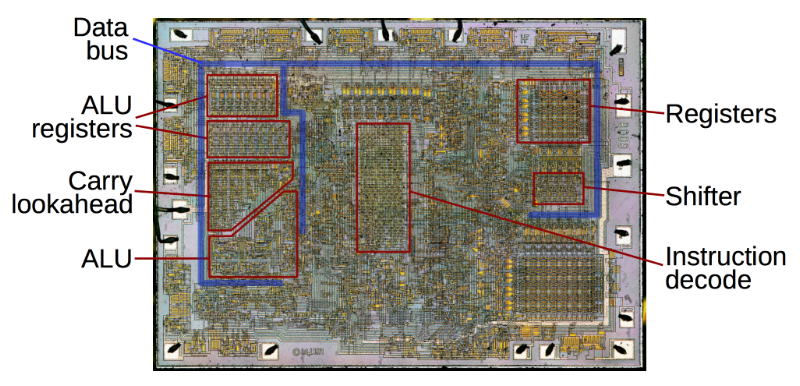Die photo of the 8008 microprocessor, showing important functional blocks.

Many parts of the chip work together to perform an arithmetic operation. First, two values are copied from the registers (on the right side of the chip) to the ALU's temporary registers (left side of the chip) via the 8-bit data bus. The ALU computes the result, which is stored back into the accumulator register via the data bus. (Note that the data bus splits and goes around both sides of the ALU to simplify routing.) The carry lookahead circuit generates the carry bits for the sum in parallel for higher performance.3 This is all controlled by the instruction decode logic in the center of the chip that examines each machine instruction and generates signals that control the ALU (and other parts of the chip).

# The Arithmetic-Logic Unit

The 8008's ALU implements four functions: Sum, AND, XOR and OR. The Sum operation adds two 8-bit numbers. The remaining three operations are standard Boolean logic operations. The AND operation sets an output bit if the bit is set in the first AND the second number. OR checks if a bit is set in the first OR the second number (or both). XOR (exclusive-or) checks if a bit is set in the first OR the second number (but not both).

The concept of carries during addition is a key part of the ALU. Binary addition in a processor is similar to grade-school long addition, except with binary numbers instead of decimal. Starting at the right, each column of two numbers is added and there can be a carry to the next column. Thus, in each column, the ALU adds two bits as well as a carry bit.

In most early microprocessors, addition of each column needs to wait until the column to the right has been added and the carry is available. The carry "ripples" through the bits, right to left, slowing the addition. The 8008, however, uses a fast carry-lookahead circuit3 to generate the carries for all 8 columns in parallel before the addition happens. Then all the columns can all be added in parallel without waiting for the carry to "ripple" through the sum. This carry-lookahead circuit is an unusual feature to see in an early microprocessor due to its complexity.

Since the 8008 is an 8-bit processor, the ALU operates on two eight-bit arguments. Most 8-bit processors (including the 8008) use a "bit-slice" construction for the ALU, with a one-bit ALU slice repeated eight times. Each one-bit ALU slice takes two input bits and the carry-in bit, and produces the output bit. In most 8-bit processors, the bit-slice ALU is arranged by stacking 8 rectangular ALU slices to form a compact, regular block. However, the 8008 has its eight ALU slices arranged in an irregular fashion—some blocks are even sideways—as shown in the diagram below. The motivation for this is that the carry lookahead circuit takes up a triangular space on the chip. To fit the remaining space better, the 8008's ALU is arranged into its unusual triangular layout.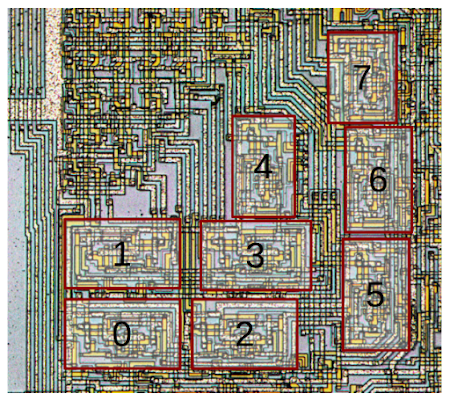Arrangement of the eight ALU slices on the 8008 microprocessor die. Unlike most processors, the 8008's ALU slices are arranged in a haphazard triangular arrangement. This fits better with the triangular carry-lookahead circuit above the ALU.

Zooming in on the die photo, we can look at one of the ALU slices and see how the circuitry is constructed. The chip is built from three layers (to simplify slightly). The topmost layer is the metal wiring. It is the most visible feature, and looks metallic (not surprisingly). In the detail below, you can see the horizontal and vertical metal traces. The polysilicon layer is underneath the metal layer and appears yellow/orange under the microscope. Polysilicon can act as wiring, but more importantly it forms the gates of the transistors, switching them on and off. The bottom layer is the grayish silicon die itself, but it is hard to see under the other layers.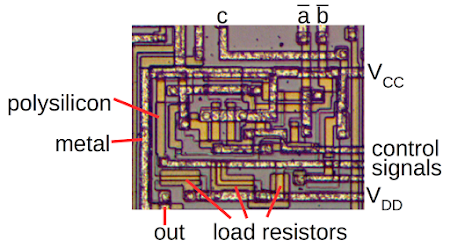Die photo of the 8008 processor, zoomed in on the circuit for one bit of the ALU.

In the diagram above, the carry c and the complemented a and b inputs enter through the metal wires at the top. The ALU output is at the bottom. The control signals are horizontal metal lines. The circuit is powered by the Vcc (+5 volts) and Vdd (-9 volts) metal lines. The brighter yellow polysilicon regions are transistors. Each gate in the circuit requires a "load resistor" connected to Vdd to pull its output low; for improved performance, these are implemented with transistors rather than resistors.

Removing the metal layer with acid makes the silicon and polysilicon layers more visible, as shown below.6 The chip is formed on a silicon wafer with regions of it "doped" with impurities to create regions of semiconducting silicon. You can see dark lines along the border between doped silicon and undoped silicon. A transistor is formed where a yellowish polysilicon wire crosses the doped silicon. The transistor forms a switch between the two silicon sides, controlled by the polysilicon gate. Each ALU slice contains 20 transistors; the diagram below points out two of them.5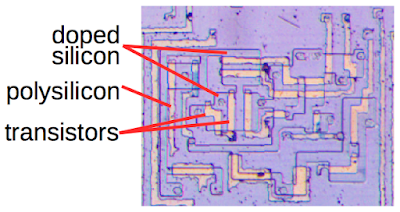With the metal layer removed from the 8008 processor die, the underlying silicon is visible. The photo shows bit 1 of the 8008's ALU.

# Simulating one slice of the ALU

By examining the die photos carefully, you can map out the ALU slice's 20 transistors and their connections. From this, you can reverse-engineer the gates that make up the circuit. I explained in my previous article how PMOS gates are structured, so I won't go into the details here. The result is the schematic below, showing one bit of the ALU. Each ALU slice takes two inputs (a and b) and the input carry c, and outputs one result bit. There are three mode lines (m1, m2 and m3) that select one of the four ALU operations.7

The schematic below is interactive. First, select an operation and the table will update with results for the eight different inputs. Next, click a row in the table, and the schematic will update, showing how the ALU computes that row. (Note that the a and b inputs to the ALU are inverted, indicated by an overbar.)

Operation:

While this ALU slice looks like it is made of many gates, physically it is only three gates: two large, multilevel AND-OR-NAND gates and one NAND gate. The AND-OR-NAND logic is implemented on the chip as a single complex gate, rather than by combining simpler gates, since a single large gate provides better performance with less circuitry than multiple small gates. One feature of MOS logic is it's just as easy to form an AND-OR-NAND gate (for instance) as a plain NAND gate.

# Understanding the ALU logic

The 8008's ALU circuit above looks like a mysterious collection of gates, but eventually I figured out the structure behind it. The starting point is a full adder that handles the Sum operation. (A full adder adds three input bits (a, b and c) and outputs the (low-order) sum bit and a carry bit.) The full adder is then heavily modified to support the logic operations, yielding the ALU from the previous section. The logic operations are implemented by using the mode lines to block parts of the circuit, yielding XOR, AND or OR, rather than the more complex Sum.

The diagram below strips down the 8008's ALU circuit to reveal the full adder "hidden" inside. The gate in red generates the carry-out from the three inverted inputs, using relatively straightforward logic. (Since the 8008 uses carry-lookahead, this carry-out signal isn't passed to the next ALU slice, but just used to generate the ALU output.) If you examine the possible sum cases, you will see that the sum bit is almost always just the carry-out inverted, except for the 0+0+0 and 1+1+1 cases. Thus, the sum bit can be generated by inverting the carry-out and handling the two exceptional cases.8 The two gates indicated below handle the exceptions by forcing the sum output to the correct value.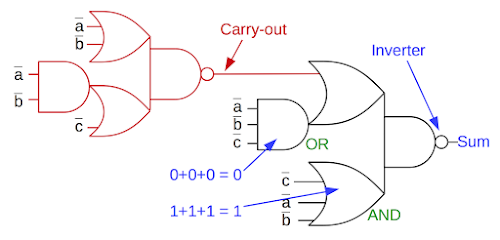Simplified 8008 ALU slice, showing the full adder circuit.

Comparing the full adder with the full ALU circuit earlier shows how the mode lines support the logic operations. Once you have a full adder, generating XOR is simply a matter of setting the carry-in to 0, which is done by the m3 control line. For the OR and AND operations, mode lines m3 and m2 respectively disable all of the circuit except the gates labeled in green.9 Thus, if you start with a full-adder and extend it to support XOR, AND and OR, the 8008's ALU circuit is a logical result.

Intel's earlier 4004 microprocessor had a simple ALU that only supported addition and subtraction, not any logic operations.10 Interestingly, the 4004's ALU circuit is almost identical to the full adder circuit shown above. So it's very likely that Intel designed the 8008 ALU by extending the 4004 ALU as described above. This would explain why the 8008's ALU generates carries internally, even though the carry lookahead circuit made this redundant.11

The 8008's ALU logic is very similar to the Z80's ALU,12 although the Z80's ALU is (surprisingly) 4 bits (details). The 8085 uses a different complex gate arrangement. The 6502 on the other hand, uses an entirely different approach: straightforward circuits for addition, AND, OR, XOR and shift-right, using pass-transistor multiplexers to select the operation.

# Instruction decoding: how the ALU knows what operation to do

The 8008 executes 8-bit instructions, which move data, perform I/O, branch, call subroutines, and so forth. The instruction decoding logic examines the instruction and determines what operation to perform, generating about 30 control signals.13 Over a quarter of the instructions perform ALU operations, and the instruction set is carefully designed so three bits of the instruction specify which of the eight operations to perform.14 By examining these bits, the instruction decoder generates the ALU's mode control lines m1, m2 and m3.

Looking at AND instructions illustrates how this works. All AND instructions have the bit pattern xx100xxx (where x is either 0 or 1). For instance, the instruction to AND with memory is 10100111 and the instruction to AND with a constant is 00100100. When the instruction decode circuit matches this pattern, it pulls the m1 control line low, which causes the ALU to perform an AND operation.7 Other bit patterns generate the other ALU control signals.15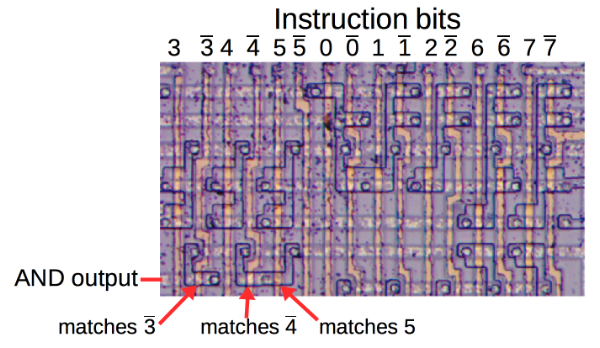Part of the 8008's instruction decode PLA. The three indicated transistors match opcode pattern XX100XXX, indicating an AND instruction.

The diagram above shows part of the instruction decode circuit. The instruction bits (and their complements) are on yellow polysilicon wires running vertically through the circuit. Each row matches a bit pattern, with a transistor connected to each instruction bit to be matched. (The doped silicon regions forming transistors are the black outlines. Circles are connections between a transistor and the row's metal line.) For example, the three transistors marked with arrows match bit 3 low, bit 4 low, and bit 5 high, detecting the AND instruction pattern. Thus, the processor uses the grid of transistors in the instruction decoder to determine the meaning of each instruction.

# Loose ends: Subtraction and rotating

The ALU implements a Sum operation, so you might wonder how subtraction is implemented. By using two's complement arithmetic, the CPU can perform subtraction by simply flipping all the bits on a value and then adding it. The ALU uses two temporary registers to hold the two operands since the ALU can't read the operands from the register file and write the result back simultaneously. One of the temporary registers has the feature that its value can be fed to the ALU directly or inverted. The subtraction instructions generate a signal causing the temporary register to provide the inverted value to the ALU, causing the ALU to perform subtraction.

One important operation in most processors is rotating or shifting the bits in a value, to the left or to the right. In most of the microprocessors I've examined, shifting is performed by the ALU.16 The 8008, on the other hand, implements the rotate logic in the register access circuit, on the opposite side of the chip from the ALU. When reading a register, the bits can be shifted one position left or right by a simple circuit before going onto the data bus.

# History of the 8008

The Intel 8008 is important historically since it is the ancestor of the dominant Intel x86 architecture that you're probably using right now.2 I wrote a detailed article for the IEEE Spectrum on early microprocessor history, so I'll just give the outline of the 8008's complicated history here.

The 8008 copies the instruction set and architecture of the Datapoint 2200, a popular minicomputer introduced in 1970 as a programmable terminal.17 As was typical for minicomputers, the Datapoint 2200 contained a CPU build from individual TTL chips, filling up a circuit board. Datapoint contracted with both Intel and Texas Instruments to build a single-chip CPU that would replace this processor board, but keeping the same architecture and instruction set.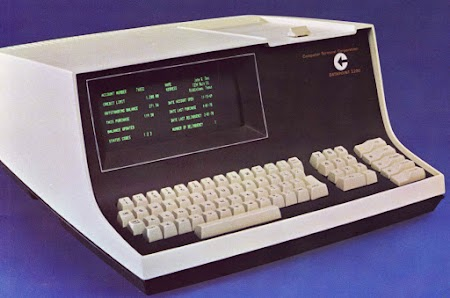The Datapoint 2200 computer. The 8008 microprocessor was built to implement the Datapoint 2200's architecture and instruction set. Photo courtesy of Austin Roche.

Texas Instruments was first to build a 2200-compatible microprocessor, creating the TMC 1795 chip. Intel got their version, the 8008, working a bit later, around the end of 1971. Datapoint rejected both processors, instead updating the Datapoint 2200 to use the 74181 TTL ALU chip. Texas Instruments couldn't find a new customer for the TMC 1795 and abandoned it. Intel, on the other hand, came up with the idea of selling the 8008 as a well-supported general-purpose processor. The 8008 led to the 8080, the 8085, 8086, and Intel's x86 line, which still retains some features of the 8008.

# Conclusion

Although the 8008 was a very early microprocessor, its ALU was more advanced than you might expect. In particular, it used a complex carry-lookahead circuit for higher performance. Unfortunately, even with the carry-lookahead circuit, the 8008 was slower than the TTL-based Datapoint 2200 processor it was supposed to replace; addition took 20µs on the 8008, compared to 16µs on the original Datapoint 2200 and just 3.2µs on the upgraded Datapoint 2200. This illustrates the speed advantage that TTL had over MOS in the early 1970s. To us, a microprocessor may seem obviously better than a board of chips, but this wasn't always the case.

If you're interested in the 8008, my previous article has a detailed discussion of the architecture, more die photos and information on how to take them, and information on semiconductor history, so take a look.

I announce my latest blog posts on Twitter, so follow me at kenshirriff. I also have an RSS feed.

# Notes and references

1. The 8008 chip was publicly announced in an article in Electronics on March 13, 1972, entitled "8-bit parallel processor offered on a single chip", offering the chips for \$200 each.

2. If you're not using an x86 processor right now, you're probably using an ARM processor. Don't feel neglected, though, since I've reverse-engineered the ARM-1 too. (Although there are many more ARM chips out there than x86, analytics show 71% of my readers are on x86.)

3. Using a carry look ahead circuit avoids the delay from a standard ripple-carry adder, where the carries propagate through the sum. The 8008's carry-lookahead is based on the Manchester carry chain, but with a separate carry chain for each carry, yielding the triangular structure you see on the die. For performance, the carry chain is implemented with dynamic logic, depending on wire capacitance, rather than with standard Boolean gates. The 74181 ALU chip in comparison, uses a different carry lookahead scheme implemented with standard logic. I plan to write more about the 8008's carry lookahead later.

4. The 8008 implements eight different arithmetic/logic functions: Add, Add with carry, Subtract, Subtract with borrow, AND, XOR, OR, and Compare.14 These are implemented in terms of the ALU's four basic operations. Subtraction is performed by inverting the second argument. The operations without carry/borrow clear the carry-in bit. Compare is simply a subtraction that doesn't store the result; it just sets the flags with the status. Thus, the four fundamental operations of the ALU are used to implement eight different arithmetic/logic operations.

5. Note that the 8008 uses PMOS transistors, rather than the faster NMOS transistors in later microprocessors such as the 8080, 6502 and Z80. If you're familiar with NMOS circuits, PMOS can be confusing since everything is backwards. PMOS transistors turn on if the gate is low, and typically pull the output high. Vdd in PMOS is negative, and "ground" is positive. The "pull-up resistor" in a PMOS gate pulls the output down. A PMOS NAND gate has transistors in parallel (compared to serial for an NMOS NAND gate). A PMOS NOR gate has transistors in serial (compared to parallel for an NMOS NOR gate).

6. The metal layer of the chip is protected by silicon dioxide passivation layer. The professional way to remove this layer is with dangerous hydrofluoric acid. Instead, I used Armour Etch glass etching cream, which is slightly safer and can be obtained at craft stores. I applied the etching cream to the die and wiped it for four minutes with a Q-tip. (Since the cream is designed for frosting glass, it only etches in spots. It must be moved around to obtain a uniform etch.) After this, I soaked the die in hydrochloric acid (pool acid from the hardware store) overnight to dissolve the metal. This was probably too long, since the edges of the polysilicon were eaten away in places.

7. The following values are used for the three mode lines to select the ALU function:

Operationm1m2m3
Sum111
And010
Or100
Xor110

8. A more straightforward way of generating the sum bit is by xoring the three inputs: a⊕b⊕c. Unfortunately, an XOR gate is relatively difficult to implement with Boolean logic, so designers will often try to avoid XOR.

9. You might wonder why the OR operation is implemented with an AND gate, and vice versa. Since the inputs and the output of the OR gate are inverted, this is equivalent to an AND gate (by De Morgan's laws), and similarly for the AND gate.

10. Strictly speaking, the 4004 microprocessor has an AU (arithmetic unit), not an ALU (arithmetic/logic unit), since it doesn't do logical operations. Since the 4004 was designed for a calculator, logical operations weren't required.

11. The 8008's full adder generates the carry-out first, and generates the sum from that. In contrast, the typical full adder circuit combines two half adders to generate the sum and carry-out separately. If the typical full adder circuit had been used in the 8008, the carry-out logic could easily be omitted.

12. To see the similarity between the Z80's ALU circuit and the 8008's, you need to swap AND and OR gates. (Apply De Morgan's laws since the 8008's ALU inputs are inverted.) In the Z80, the carry-out comes from the ALU rather than a carry-lookahead circuit, so the control lines are somewhat different. But the fundamental ALU circuit is otherwise the same between the 8008 and Z80, which is not surprising since Federico Faggin worked on both chips.

13. Instruction decoding is based on a Programmable Logic Array (PLA), an arrangement of transistors that efficiently implements logic gates. These gates match bit patterns and generate the appropriate control signals for the rest of the chip. The 8008's PLA has 16 input lines flow vertically through the PLA. Each row in the PLA matches a bit pattern and generates a control signal output.

In more detail, each row output line is pulled low by a load resistor/transistor to Vdd. The transistors are connected between the row line and Vcc (+5V). The bit lines are connected to the transistor's gate. If any bit line is low (indicating a mismatch), the PMOS transistor turns on, pulling the row line high. Thus, if there is no mismatch, the control line is low, and if there is a mismatch, the control line is high. In other words, each row is a NAND gate with instruction bit inputs.

The input lines are ordered as follows: bit 3, bit 3 complement, 4, 4', 5, 5', 0, 0', 1, 1', 2, 2', 6, 6', 7, 7'. This order may seem strange, but there's a reason for it. In the 8008, the ALU operation is selected by bits 3, 4 and 5 of the instruction. By putting those bits on the left side of the PLA, they are closer to the ALU. Some rows of the PLA actually decode two instructions: bits 3, 4 and 5 are decoded on the left side, generating an ALU control signal, while the remaining bits are decoded on the right side generating a different control signal. This increases the PLA density and saves space on the chip.

14. The 8008's instruction set is designed around octal. Among other things, there are 8 ALU operations, 8 registers and 8 conditionals. In octal, the ALU instructions have the value 2ar, where a is the ALU operation to perform (0 through 7) and r is the register to use (0 through 7, where 7 indicates memory). The octal structure originates with the Datapoint 2200, which decoded instructions with TTL 7442 BCD chips that decoded groups of three bits. This octal structure persisted in descendants of the 8008, including the Z80 and x86. Unfortunately, these instruction sets are almost always presented in hexadecimal, which hides the underlying structure.

15. The instruction decoder generates all the signals required by the ALU. As described above, AND matches xx100xxx, pulling the m1 control signal low. An OR opcode has the bit pattern xx110xxx, which causes the instruction decode circuit to pull the m2 control line low. An XOR instruction has the bit pattern xx101xxx. The m3 control line is pulled low for patterns xx10xxxx or xx1x0xxx, matching AND, OR or XOR instructions. The subtract (with and without borrow) instructions match xx01xxxx, generating a signal that inverts the second argument.

16. Different processors use a variety of techniques for shifting. In the Z80, shifting is performed as data enters the ALU. The 6502 performs a left shift with "A plus A", and has a path inside the ALU for right shifts; the 8085 is similar. The ARM-1 has a barrel shifter next to the ALU that performs arbitrary shifts.

17. The instruction set of the Datapoint 2200 is described in the Reference Manual. The 8008 has a couple minor changes. For instance, the 8008 has increment and decrement instructions that are not present in the 2200.